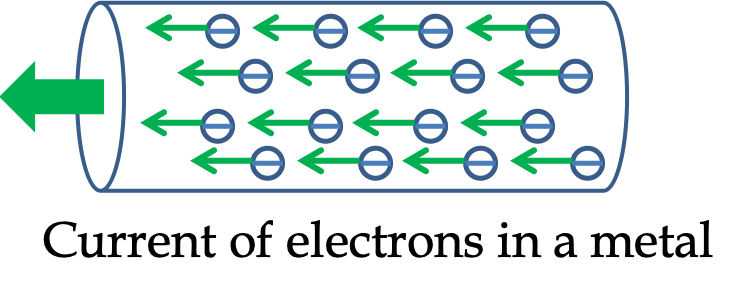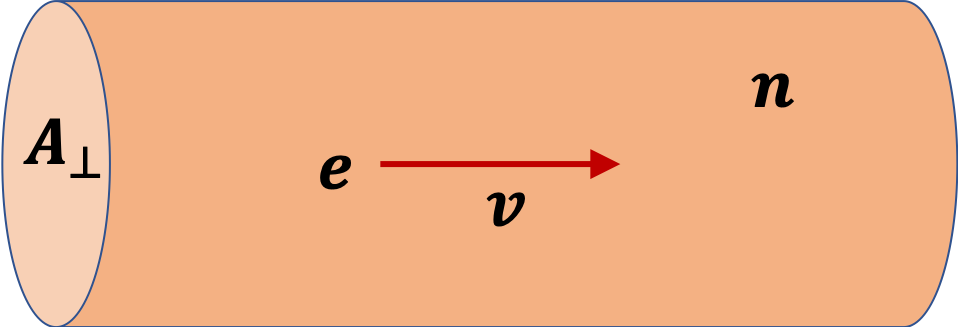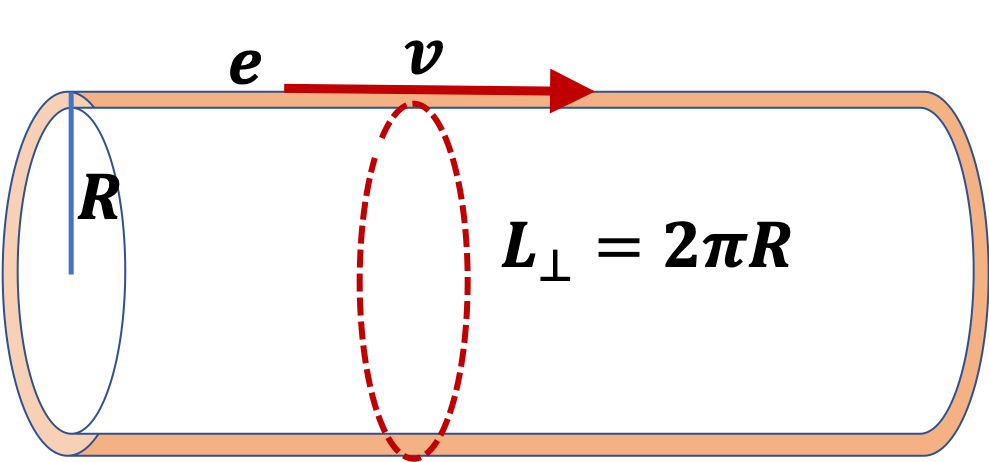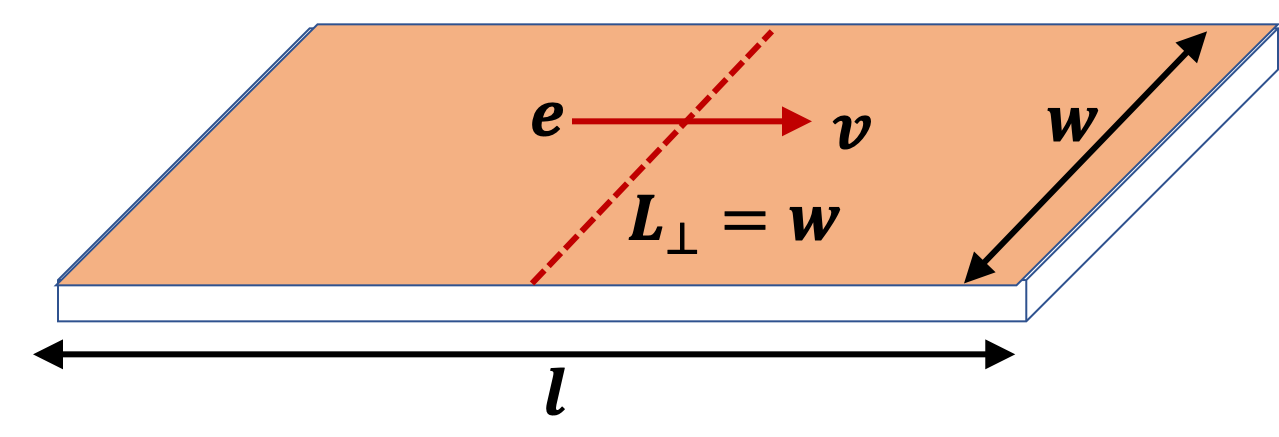## Section35.1Electric Current

The flow of charges produces an electric current just as the flow of water produces a water current. While water current is quantified by the amount of water that flows per unit time through the cross-section of flow, electric current is quantified by total charge flowing per unit time through the cross-section of flow.

SI unit of current would be $\text{C/s}\text{,}$ which is also called ampere, which is denoted as $\text{A}$ and $\text{amp}\text{.}$

In metals, current flow occurs by moving conduction electrons. In semiconductors, current flow may occur either by moving conduction electrons or holes. In electrolytic cells, current flow occurs by both positive and negative ions.Figure 35.1.1. Examples of electric current. Electric current in a metal wire is carried by conduction electrons. Electric current in an electrolytic solution is carried by both positive and negatively charged ions.

### Subsection35.1.1Electric Current in a Metal Wire

Let's look at current flowing in a thin wire of cross-section area $A_\perp\text{.}$

Let $v$ be the speed with which electrons are drifting in the wire. We will make an assumption that all drifting electrons have the same speed. Let $n$ be number of conduction electrons per unit volume.Since, each electron carries a charge $q=-e\text{,}$ the charge per unit length of wire in these conduction electrons is

\begin{equation*} \lambda_e = -nA_\perp e. \end{equation*}

Then, we will find that $-neAv$ amount of charge flows per unit time. This is electronic current in the wire.

\begin{equation} I_\text{electron} = -neA_\perp v.\tag{35.1.1} \end{equation}

But, the convention of electric current is to leave the sign out. Just ignoring the sign will give us wrong direction of charge flow. So, if we ignore the sign, we will also need to flip the direction of flow. Therefore, in metal, we say that electric current is

\begin{equation} I = neA_\perp v,\tag{35.1.2} \end{equation}

which is in the opposite direction in which electron is drifting.

### Subsection35.1.2Current Density

The quantity $I/A_\perp$ gives current per unit cross-section area. It is called current density and denoted by letter $J\text{.}$

\begin{equation} J = \dfrac{I}{A_\perp} = n e v.\tag{35.1.3} \end{equation}

Current density is useful when studying situations where current density varies over the cross-section. For instance, when current is flowing in a layered material with different conductivities, $J$ will be different for different material.

Suppose current density $J$ is same through a cylindrical wire of radius $R\text{.}$ What will be current in the center upto a radius $R/2$ and what will be current in the shell of inner radius $R/2$ and outer radius $R\text{?}$ Let's call the first one as $I_\text{center}$ and the other one as $I_\text{edge}\text{.}$

\begin{align*} I_\text{center} \amp = \pi J (R/2)^2 = \frac{1}{4} \pi J R^2,\\ I_\text{edge} \amp = \pi J ( R^2 - (R/2)^2 ) = \frac{3}{4} \pi J R^2. \end{align*}

Clearly, current for the same thickness is higher near the edge than near the center. You might say that in a wire, most of the current flows at the edge than at the center.

For nonsteady currents, such as alternating current, current density varies across the cross-section. At high frequencies, current density is non-zero only near the surface, upto a thickness, called the skin depth.

### Subsection35.1.3Surface Current Density

Current on metals flows mostly on the surface. In this case cross-section of flow is a line, whose length will be denoted by $L_\perp\text{.}$ Therefore, in place of current density, $J\text{,}$ we use surface current density, denote by $K\text{.}$

\begin{equation} K = \dfrac{I}{L_\perp}.\tag{35.1.4} \end{equation}

In terms of drift speed $v$ and number of electrons per unit surface area, $n^\prime\text{,}$ this becomes

\begin{equation} K = n^\prime e v.\tag{35.1.5} \end{equation}

In case of a metal wire of radius $R\text{,}$ the cross-section length will be

\begin{equation*} L_\perp = 2\pi R. \end{equation*}In the case of a metal sheet of length $l$ and width $w\text{,}$ with current flowing along the length, the cross-sectional length will be the width,

\begin{equation*} L_\perp = w. \end{equation*}### Subsection35.1.4Vector Current Density

In some instances it is useful to define vector current densities, $\vec J$ and $\vec K$ by incorporating the drift velocity vector, $\vec v\text{.}$ The vector (volume) current density $\vec J$ for current in a metal will be in the opposite direction of the direction in which electron drifts since the charge on electron is negative.

\begin{equation*} \vec J = -ne\vec v, \end{equation*}

where $n$ is number density of conduction electrons, i.e., number of conduction electrons per unit volume. Similarly, the vector surface surrent density will be

\begin{equation*} \vec K = -n^\prime e \vec v, \end{equation*}

where $n^\prime$ is number surface density of conduction electron, i.e., number of conduction electrons per unit surface area.

How many electrons are present in 1 gram samples of (a) Copper, (b) Gold, and (c) Aluminum? Atomic weights: Cu 63.5, Au 197, Al 27; Atomic numbers Cu 29, Au 79, Al 13.

Hint

Use Avogadro number $N_A$ atoms in a mole.

(a) $2.75\times 10^{23}\text{,}$ (b) $2.41\times 10^{23}\text{.}$

Solution

These exercises are solved by noting that there are Avogadro number of atoms in one atomic weight of an element expressed in grams. The atomic number is equal to the number of electrons in one neutral atom. Let $N_{\text{Cu}}\text{,}$ $N_{\text{Au}}\text{,}$ and $N_{\text{Al}}$ ne the number of electrons in one gram each of copper, gold and aluminum. Therefore,

(a)

\begin{align*} N_{\text{Cu}} \amp = \dfrac{29\times 6.022\times 10^{23}\:\text{mole}^{-1}}{63.5\:\text{g.mole}^{-1}}\\ \amp = 2.75\times 10^{23}. \end{align*}

(b)

\begin{align*} N_{\text{Au}} \amp = \dfrac{79\times 6.022\times 10^{23}\:\text{mole}^{-1}}{197\:\text{g.mole}^{-1}}\\ \amp = 2.41\times 10^{23}. \end{align*}

(c)

\begin{align*} N_{\text{Al}} \amp = \dfrac{13\times 6.022\times 10^{23}\:\text{mole}^{-1}}{27\:\text{g.mole}^{-1}}\\ \amp = 2.90\times 10^{23}. \end{align*}

In a thin wire of copper $20\text{ mA}$ of current is flowing. How many electrons are passing through through the wire every minute?

Hint

Convert charge into number of electrons.

$7.5\times 10^{18}\text{.}$

Solution

We use the definition of current of $1\text{ A}$ as $1\text{ C/s}$ and convert the charge into number of electrons. Hence,

\begin{align*} I \amp = 20\times 10^{-3}\:\dfrac{\text{C}}{\text{s}}\times \dfrac{1\:\text{e}}{1.6\times 10^{-19}\:\text{C}}\times \dfrac{60\:\text{s}}{1\:\text{min}} \\ \amp = 7.5\times 10^{18}\:\dfrac{\text{e}}{\text{min}}. \end{align*}

Find drift speed of conduction electrons in a cylindrical copper wire of diameter $4\text{ mm}$ that carries a $10\text{ A}$ current.

(a) Find number density of conduction electrons.

(b) Find the drift speed of electrons.

(c) What would be the drift speed if the wire carrying $10\text{ A}$ current was $0.4\text{ mm}\text{,}$ thick, i.e. one-tenth as thick?

Data about Cu: one electron per atom as conduction electron, density = $8,960 \text{ kg/m}^3\text{,}$ atomic weight = $0.063546 \text{ kg/mole}\text{,}$ atomic number = $29\text{.}$

Data: Avogadro number = $6.022\times 10^{23}\text{.}$

Hint

(a) Find number of atoms per unit volume. (b) use formula of current.

(a) $8.49\times 10^{28}\text{ m}^{-3}\text{,}$ (b) $5.84\times 10^{-5}\text{ m/s} \text{,}$ (c) $5.84\times 10^{-3}\text{ m/s}\text{.}$

Solution 1 (a)

(a) When we divide density by atomic weight, we will get number of moles per unit volume. Let's denote that by $\mu\text{.}$

\begin{equation*} \mu = \dfrac{8,960 \text{ kg/m}^3}{0.063546 \text{ kg/mole}} = 141,000\text{ mole/m}^3. \end{equation*}

There are Avogador number of atoms in one mole. And since each atom contributes one electron to conduction, the number of these electrons per unit volume will be same as number of atoms per unit volume.

\begin{equation*} n = 141,000\text{ mole/m}^3 \times 6.022\times 10^{23}\text{ mole}^{-1} = 8.49\times 10^{28}\text{ m}^{-3}. \end{equation*}
Solution 2 (b)

(b) The area of cross-section is

\begin{equation*} A = \pi r^2 = \pi \times0.002^2 = 1.26\times 10^{-5}\text{ m}^2. \end{equation*}

Therefore, drift speed is

\begin{align*} v \amp = \dfrac{I}{n A e}\\ \amp = \dfrac{10\text{ A}}{8.49\times 10^{28}\text{ m}^{-3} \times 1.26\times 10^{-5}\text{ m}^2 \times 1.6\times 10^{-19}\text{ C} } \\ \amp = 5.84\times 10^{-5}\text{ m/s}. \end{align*}
Solution 3 (c)

(c) Since drift speed is inversely proportional to $A_\perp\text{,}$ we will just scale the result in (b) to get $v$ here.

\begin{equation*} v_c = v_b\times \dfrac{A_b}{A_c} = v_b \times 10^2 = 5.84\times 10^{-3}\text{ m/s}. \end{equation*}

A steady current of $2\text{ A}$ flows through a gauge number 12 wire ($0.0808\text{-in}$ diameter) made of copper. (a) Find the current density. (b) How many conducting electrons are there in one inch of the wire? (c) How many total electrons are there in a one-inch of the wire? (d) Find the drift speed of electrons.

Hint

Start out by converting into SI units.

(a) $6.05\times 10^{5}\ \text{A/m}^2\text{,}$ (b) $1.44\times 10^{22}\text{,}$ (c) $4.17\times10^{23}\text{,}$ (d) $4.85\times 10^{-5}\ \text{m/s}\text{.}$

Solution 1 (a)

(a) Let us convert the diameter from inch into meter first.

\begin{equation*} D = 0.0808\:\text{in}\times \dfrac{0.0254\:\text{m}}{1\:\text{in}} = 2.05\times 10^{-3}\:\text{m}. \end{equation*}

The area of cross-section,

\begin{equation*} A = \dfrac{\pi}{4}\:D^2 = 3.31\times 10^{-6}\:\text{m}^2. \end{equation*}

Hence, the current density is

\begin{equation*} J = \dfrac{I}{A} = \dfrac{2\:\text{A}}{3.31\times 10^{-6}\:\text{m}^2} = 6.05\times 10^{5}\:\text{A}/\text{m}^2. \end{equation*}
Solution 2 (b)

(b) The number of mobile electrons in one inch of the wire can be obtained from the number of atoms of copper since each atom contributes one electron to the conduction.

\begin{align*} \text{No. of cond. electrons} \amp = \text{No. of atoms}\times \text{No. of cond. electrons/atom} \\ \amp = \text{No. of atoms}\times 1 = \text{No. of atoms}. \end{align*}

Now, the number of atoms in one inch of copper wire will be

\begin{align*} N_{\text{atoms}} \amp = \text{Volume} \times \text{Density} \times \dfrac{1}{\text{At. Wt.}}\times \text{Avo. No}.\\ \amp = (2.54\:\text{cm}\times 0.0331 \:\text{cm}^2) \times 8.23\:\dfrac{\text{g}}{{\text{cm}}^3} \times \dfrac{1}{29\:\text{g}}\times 6.022\times 10^{23}\\ \amp = 1.44\times 10^{22}. \end{align*}
Solution 3 (c)

(c) There are 29 times as many electrons in copper as there are conducting electrons.

\begin{equation*} \text{Total} = 29\times 1.44\times 10^{22} = 4.17\times10^{23}. \end{equation*}
Solution 4 (d)

(d) The drift speed of conducting electrons is related to the conduction electron density and current density.

\begin{equation*} J = e n v. \end{equation*}

The density of conduction electrons can be found from the number of electrons in the volume within one-inch length we calculated above.

\begin{equation*} n = \dfrac{1.44\times 10^{22}}{2.54\:\text{cm}\times 0.0331 \:\text{cm}^2} = 1.71 \times 10^{23}\:\text{cm}^{-3}. \end{equation*}

Hence,

\begin{align*} v \amp = \dfrac{J}{e n} \\ \amp = \dfrac{6.05\times 10^{5}\:\text{A}/\text{m}^2}{1.6\times 10^{-19}\:\text{C}\times 1.71 \times 10^{27}\:\text{m}^{-3}} = 22\:\text{m/s}. \end{align*}

How much current flows if the current density on the surface of an aluminum plate of width $3 \text{ cm}$ is $20 \text{ A/m}\text{?}$

Hint

Use definition of surface current density.

$0.6 \text{ A}\text{.}$

Solution

This is an exercise in using the definition of surface current density $K\text{.}$

\begin{equation*} I = K\:L_\perp = 20\:\dfrac{\text{A}}{\text{m}}\times 0.03\:\text{m} = 0.6\:\text{A}. \end{equation*}

A current of $10 \text{ A}$ passes through a cylindrical copper pipe of inner radius $2 \text{ cm}$ and outer radius $2.5 \text{ cm}\text{.}$ Find the current density.

Hint

Use definition of $J\text{.}$

$1.4\times 10^{4} \ \text{A/m}^2\text{.}$

Solution

This is an exercise in using the definition of volume current density J.

\begin{align*} J \amp = \dfrac{I}{A_\perp} \\ \amp = \dfrac{10\:\text{A}}{\pi\left( 0.025^2 - 0.02^2 \right)\:\text{m}^2}\\ \amp = 1.4\times 10^4\:\text{A/m}^2. \end{align*}

A copper pipe of inner radius $1.5 \text{ cm}$ and outer radius $1.8 \text{ cm}$ surrounds a copper rod of rod of radius $0.5 \text{ cm}\text{.}$ A $5 \text{ A}$ current flows in the pipe in one direction and the same amount flows in the inner rod in the opposite direction. Write the current density as a function of distance from the center.

Hint

Use $z$ axis along the current in the rod and work with $J_z\text{.}$

$J_{z,\text{rod}} = 6.37\times 10^4 \ \text{A/m}^2\text{,}$ $J_{z,\text{shell}} = -1.61\times 10^4 \ \text{A/m}^2\text{.}$

Solution

The current density is non-zero only in the material. The current density in the rod and the shell are related to the cross-sectional ares of the material in which current flow. Furhtermore, directions of flow in the two are opposite. Let $+z$ axis be the direction of current in the rod, then $-z$ wil be the direction in the shell. We can then write the $z$ component of vector current density.

\begin{align*} J_{z,\text{rod}} \amp = \dfrac{I}{A_{\text{rod}}}\\ \amp = \dfrac{5\:\text{A}}{\pi\times 0.005^2\:\text{m}^2} = 6.37\times 10^4\:\text{A/m}^2,\\ J_{z,\text{shell}} \amp = \dfrac{I}{A_{\text{shell}}}\\ \amp = \dfrac{5\:\text{A}}{\pi\times (0.018^2-0.015^2)\:\text{m}^2} = 1.61\times 10^4\:\text{A/m}^2. \end{align*}

Let $s$ denote the distance of a point from the axis of the cylinder. Then the $J_z(s)\text{,}$ current density as a function, will be

\begin{equation*} J_z = \begin{cases} 6.37\times 10^4\:\text{A/m}^2, \amp s \le 0.5\:\text{cm}\\ - 1.61\times 10^4\:\text{A/m}^2, \amp 1.5\:\text{cm} \le s \le 1.8\:\text{cm} \end{cases} \end{equation*}

A steel cylindrical rod of radius $5 \text{ cm}$ carries $30 \text{ amps}$ of current uniformly spread through out its cross section. (a) How much current flows within $1 \text{ cm}$ of its center? (b) How much current flows between $2 \text{ cm}$ and $3 \text{ cm}$ of its center? (c) How much of the current flows between $4 \text{ cm}$ and $5 \text{ cm}$ of its center?

Hint

Use $I = J A\text{.}$

(a) $1.2\text{ A}\text{,}$ (b) $6\text{ A}\text{,}$ (c) $10.8\text{ A}\text{.}$

Solution

Since the current is uniformly distributed throughout the cross-section, the current density is not dependent on the distance from the axis and found to be

\begin{equation*} J = \dfrac{I}{A} = \dfrac{30\:\text{A}}{\pi\times 0.05^2\:\text{m}^2} = 3.82\times 10^3\:\text{A/m}^2 \end{equation*}

Now we use the current density to solve different parts of the problem.

(a) Only the current passing through the area of cross-section matters.

\begin{equation*} I = J\:A = 3.82\times 10^3\:\text{A/m}^2 \times (\pi\times 0.01^2\:\text{m}^2) = 1.2\:\text{A}. \end{equation*}

(b) Between 2 cm and 3 cm, the area of cross-section is different.

\begin{equation*} I = J\:A = 3.82\times 10^3\:\text{A/m}^2 \times [\pi (0.03^2 - 0.02^2 )\:\text{m}^2] = 6\:\text{A}. \end{equation*}

(c) Between 4 cm and 5 cm the area of cross-section is even bigger.

\begin{equation*} I = J\:A = 3.82\times 10^3\:\text{A/m}^2 \times [\pi (0.05^2 - 0.04^2 )\:\text{m}^2] = 10.8\:\text{A}. \end{equation*}

More exercise for you: Find the current through 1cm-2cm and 3cm-4cm and add up all the current to show that the total current is indeed 30 A.

A 4-gauge wire of a special material of diameter $0.2038\text{ in}$ carries $10\text{ amp}$ current with a non-uniform current density across the cross-section of the wire. The current density increases linearly with distance from the center, $J = a s\text{,}$ with constant $a=5\text{ A/m}^3$ and $s$ is the distance from the axis of the wire in m. Find current flowing within $0.1\text{ mm}$ of the outer surface, and compare it with the current that flows within $0.1\text{ mm}$ of the center.

Hint

Use $I = \int J dA$ for the appropriate area.

$9.42\text{ A versus } 1.73\text{ A}\text{.}$

Solution

Let us first convert radius $R$ of the wire in metric unit.

\begin{equation*} R = \dfrac{0.2038\:\text{in}}{2} = 2.59\times 10^{-3}\:\text{m}. \end{equation*}

The relation between the current density and total current can be used to find the constant $a\text{.}$

\begin{equation*} \int\: J\:dA \ \ \Longrightarrow\ \ \int\: J\: 2\pi s ds\ \ \Longrightarrow\ \ 2\pi a\int_0^R\: s^2\: ds = I. \end{equation*}

Hence,

\begin{equation*} a = \dfrac{3 I}{2\pi R^3} = \dfrac{3\times 10\:\text{A}}{2\pi (2.59\times 10^{-3}\:\text{m})^3} = 2.75\times 10^{8}\:\text{A/m}^3. \end{equation*}

The current flowing within 0.1 mm of the outer surface is found by integrating $J$ there.

\begin{equation*} I = 2\pi a \int_{R-d}^R\: s^2\: ds = \dfrac{2\pi a}{3}\:\left[ R^3 - (R-d)^3 \right]= 9.42\:\text{A}. \end{equation*}

The current flowing within 0.1 mm of the axis has different limits on the integral.

\begin{equation*} I = 2\pi a \int_0^d\: s^2\: ds = \dfrac{2\pi a}{3}\:d^3 = 1.73\:\text{A}. \end{equation*}

A 10-gauge wire of a special material diameter $0.102 \text{in}$ carries $10 \text{amp}$ current with a non-uniform current density. The current density increases with distance from the center as given by $J=\frac{a}{b^2+s^2}\text{,}$ with constant $a= 10 \text{ A}\text{,}$ $b=0.1 \text{ m} \text{,}$ and $s$ is the distance from the axis of the wire in $\text{m}\text{.}$ Find current flowing within $0.1 \text{ mm}$ of the outer surface, and compare it with the current that flows within $0.1 \text{ mm}$ of the center.

Hint

Use $I = \int J dA$ for the appropriate area.

$0.7687\:\text{A}\text{,}$ $0.7697\:\text{A}\text{.}$

Solution

Let us first convert the radius $R$ of the wire in metric unit.

\begin{equation*} R = \dfrac{0.102\:\text{in}}{2} = 1.3\times 10^{-3}\:\text{m}. \end{equation*}

The relation between the current density and total current can be used to find the constant $a\text{.}$

\begin{equation*} \int\: J\:dA \ \ \Longrightarrow\ \ \int\: J\: 2\pi s ds\ \ \Longrightarrow\ \ 2\pi a\int_0^R\: \dfrac{s\: ds}{s^2+1} = I. \end{equation*}

Hence,

\begin{equation*} a = \dfrac{I}{\pi\:\ln(R+1)} = \dfrac{10\:\text{A}}{\pi\times \ln(1.0013)} = 2450\:\text{A}. \end{equation*}

The current flowing within 0.1 mm of the outer surface is found by integrating $J$ there.

\begin{equation*} I = 2\pi a \int_{R-d}^R\: \dfrac{s\: ds}{s^2+1} = \pi a \ln\left( \dfrac{R+1}{R-d-1} \right) = 0.7687\:\text{A}. \end{equation*}

The current flowing within 0.1 mm of the axis has different limits on the integral.

\begin{equation*} I = 2\pi a \int_0^d\: \dfrac{s\: ds}{s^2+1}= \pi a \ln\left( d+1\right) = 0.7697\:\text{A}. \end{equation*}